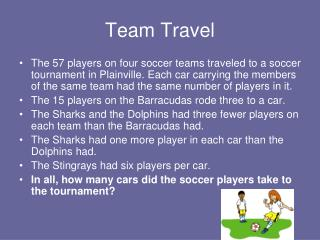DownloadDownload PresentationTeam Travel

# Team Travel

Télécharger la présentation## Team Travel

- - - - - - - - - - - - - - - - - - - - - - - - - - - E N D - - - - - - - - - - - - - - - - - - - - - - - - - - -
##### Presentation Transcript

1. Team Travel • The 57 players on four soccer teams traveled to a soccer tournament in Plainville. Each car carrying the members of the same team had the same number of players in it. • The 15 players on the Barracudas rode three to a car. • The Sharks and the Dolphins had three fewer players on each team than the Barracudas had. • The Sharks had one more player in each car than the Dolphins had. • The Stingrays had six players per car. • In all, how many cars did the soccer players take to the tournament?

2. Answer • Barracudas15 players ÷ 3 = 5 cars • Sharks12 players ÷ 4 = 3 cars • Dolphins12 players ÷ 3 = 4 cars • Stingrays18 players ÷ 6 = 3 cars • Add the number of cars for each team to find out the total number of cars the teams took to the soccer tournament.     5 + 3 + 4 + 3 = 15; 15 cars in all

3. Adding and Subtracting Mixed Numbers Lesson 6-3

4. Review - Adding and Subtracting Fractions with Like Denominators • To add or subtract fractions with like denominators, add or subtract the numerators and write the result over the denominator. Simplify the fraction. 1 5 3 5 4 5 The denominator always stays the same. + =

5. Review - Adding and Subtracting Fractions with Unlike Denominators • Find the LCD, rename the fractions. Then add or subtract with like denominators. 7 8 5 6 8 6 2 4 3 2 x 4 x 3 = 24 LCD 21 24 20 24 1 24 20 7 8 21 5 6 = 24 24

6. Adding and Subtracting Mixed Numbers • First add or subtract the fractions. If necessary, rename the fractions with the LCD. Then add or subtract the whole numbers and simplify. 4 9 2 9 6 9 2 3 7 10 17 17 + = =

7. Try Some More 5 6 1 3 6 3 3 _ 8 2 = 2 1 LCD = 6 5 6 2 6 3 6 1 2 _ 8 2 6 6 = =

8. Try Some More 5 9 1 6 9 6 3 1 + 4 = 3 2 LCD = 18 10 18 3 18 13 18 + 1 4 5 =

9. Your Turn 1 12 7 12 2 3 + 3 14 17 = 7 10 3 5 1 10 - 9 4 5 =

10. Another Example 5 9 2 3 9 3 - 3 11 8 3 1 LCD = 9 5 9 6 9 Borrow from the whole number, to get a larger numerator. - 11 8 5 9 14 One whole is equal to 9 pieces. Add 9 + 5 to get the new numerator. 10 11 9

11. Another Example 8 9 2 3 14 = 10 - 2 8 9

12. Another way to look at it… 1 4 3 3 wholes

13. Another way to look at it… 1 4 3 5 pieces 2 wholes 1 4 5 4 Can be renamed as… 3 2

14. Another Example 3 8 7 8 10 8 Rename the improper fraction. + 2 5 7 = 1 2 8 1 4 2 8 8 10 8 8 1 + 7 = = 8 2

15. Practice / Homework • Practice: Text pg. 250 # 15-22 • Homework: Lesson 6-3 # 2-24 evens • Quiz Friday: Lessons 6-1, 6-2, 6-3 (Estimating fractions, adding and subtracting fractions and mixed numbers)